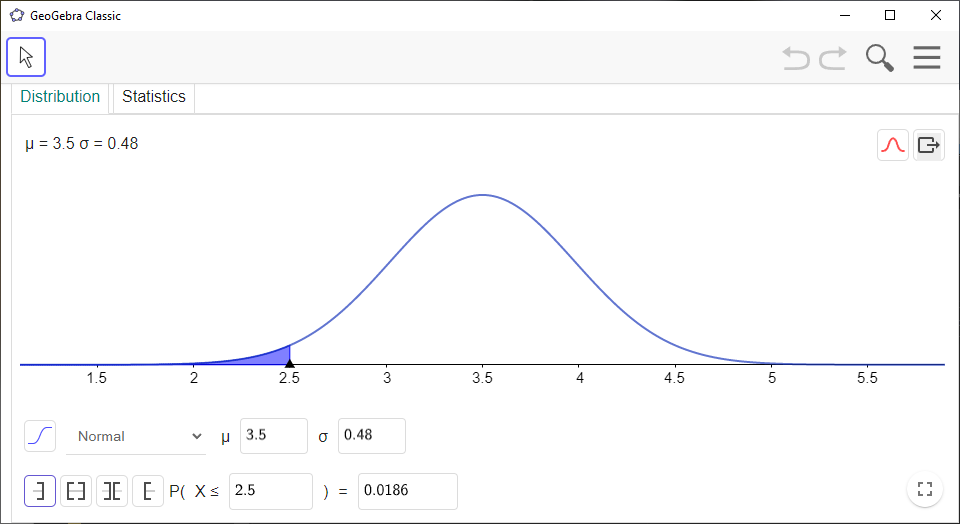# How to Use GeoGebra as a Normal Distribution Calculator

Here’s how you can use the `Probability Calculator`in `GeoGebra` to compute probabilities from the normal distribution:

`GeoGebra` Instruction 1

1.
Open `Probability Calculator`under`View` in`Menu`.
2.
Make sure that you choose the `Distribution` tab at the top of the window.
3.
Select `Normal` from the drop-down list. Enter the values of $\mu$ and $\sigma$ from your problem.
4.
Choose `Left-sided`, `Interval`, `Two tailed`, or `Right-sided`depending on you problem.